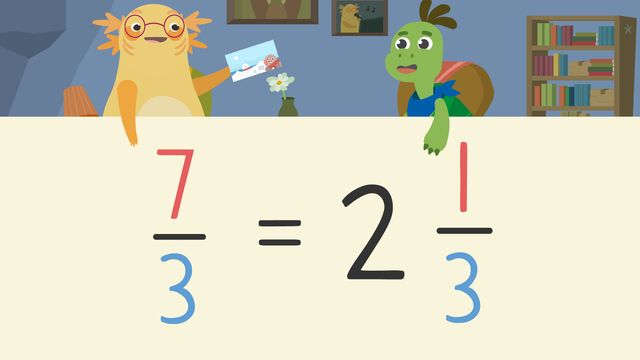# Improper Fractions and Mixed NumbersRating

Ø 4.0 / 5 ratings
The authorsTeam Digital
Improper Fractions and Mixed Numbers
CCSS.MATH.CONTENT.4.NF.B.3.C

## Converting Improper Fractions to Mixed Numbers

Sometimes, you need to convert improper fractions to mixed numbers. Let’s read about converting improper fractions to mixed numbers in this text. What is an Improper Fraction and What is a Mixed Number? There are three types of fractions:

Type Description
Proper fraction The numerator is smaller than the denominator.
Improper fraction The numerator is larger than the denominator.
Mixed number A combination of a whole number and a fraction.

Can you convert improper fractions to mixed numbers? The answer is yes, you can change improper fractions to mixed numbers. The next section will explain how to change improper fractions to mixed numbers using simple steps.

## Converting Improper Fractions to Mixed Numbers – Steps

How do you convert an improper fraction to a mixed number? We can convert, or change, improper fractions to mixed numbers so they are easier to understand. Here is an example of how to make improper fractions into mixed numbers:

• Step 1: Represent seven-thirds using fraction bars.• Step 2: Count how many wholes we have shaded in and write the whole number.• Step 3: Record the remaining part as a fraction.If you look closely at the image above you can see how to write improper fractions as mixed numbers. You will find that the answer is the mixed number is two and one third, once you follow the steps on how to turn improper fractions into mixed numbers.

## Converting Improper Fractions to Mixed Numbers – Summary

How do you change improper fractions to mixed numbers? Remember, these steps explain how to turn improper fractions to mixed numbers:

Step What to do
1 Represent the fraction using fraction bars.
2 Count how many wholes we have shaded in
and write the whole number.
3 Record the remaining part as a fraction.

Now, can you change improper fractions to mixed numbers? Practice how to convert improper fractions to mixed numbers on this website with the improper fractions to mixed numbers worksheet along with other activities, and exercises.

## Frequently Asked Questions regarding Fractions and Mixed Numbers

What is an Improper Fraction and What is a Mixed Number?
Can you convert improper fractions to mixed numbers?
How do you convert an improper fraction to a mixed number?

## Improper Fractions and Mixed Numbers exercise

Would you like to apply the knowledge you’ve learned? You can review and practice it with the tasks for the video Improper Fractions and Mixed Numbers.
• ### What are improper fractions?

Hints

The numerator is the top part of the fraction, the denominator is the bottom part of the fraction.

Here is an example of a mixed number. What do you notice about it?

Solution
• An improper fraction is a fraction with a numerator that is greater than the denominator.
• A mixed number has a combination of a whole number and a fraction.
• 3$\frac3 4$ is a mixed number because it has a whole number and a fraction.
• $\frac8 3$ is an improper fraction because the numerator is greater than the denominator.
• ### Sea stew ingredients.

Hints

Convert the improper fraction by drawing a fraction bar. The denominator is how many parts the whole is broken in to.

Count up the whole fraction bars that have been completely shaded, this is the whole number part of your mixed number.

After you have counted the whole bars, how many extra parts are shaded? This will be your numerator.

Solution

$\frac7 5$ is equal to 1$\frac2 5$

$\frac5 3$ is equal to 1$\frac2 3$

$\frac{12} {5}$ is equal to 2$\frac2 5$

$\frac7 3$ is equal to 2$\frac1 3$

• ### Where are the errors?

Hints

Try drawing each improper fraction using fraction bars. Remember that the denominator shows how many parts each bar should have.

If all the parts of a fraction bar have been shaded, this is equal to one whole.

Solution

Here are the numbers that you should have highlighted.

The correct answers to each are shown.

• ### Ordering fractions and mixed numbers.

Hints

Begin by converting the improper fractions to mixed numbers. Or convert all the fractions to the same denominator, like fourths.

Can your fraction be simplified for example, $\frac3 6$ is equal to $\frac1 3$?

When using a fraction bar, remember that the denominator tells you how many parts the fraction bar is split into.

Solution

The fractions in order of size from smallest to greatest are:

• $\frac{10} {8}$ (This is equivalent to $\frac5 4$ which is 1$\frac1 4$)
• 1$\frac1 2$ (This is equivalent to 1$\frac2 4$)
• $\frac7 4$ (This is equivalent to 1$\frac3 4$)
• $\frac9 4$ (This is equivalent to 2$\frac1 4$)
• $\frac6 2$ (This is equivalent to 3)
• ### Using fraction bars.

Hints

Look at the denominator (the bottom part of the fraction), this tells you how many parts the fraction is split into.

Look at the numerator (the top part of the fraction), this tells you how many parts are shaded in.

Solution

• ### Improper, mixed and whole numbers.

Hints

Can the fraction be simplified, for example, $\frac3 6$ can be simplified to $\frac1 2$.

Convert the improper fractions to mixed numbers by drawing a fraction bar, use the denominator to decide how many parts the bar needs.

Count how many parts are shaded in total, this is the numerator.

Solution